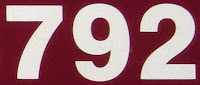## Monday, February 8, 2010

### 792

792 = 23 x 32 x 11.

792 is 1100011000 in base 2 (binary) and 2211 in base 7.

792 is the number of integer partitions of 21.

792 is a number that can be expressed as the difference of the squares of primes in exactly four distinct ways.792 is the number of inches in a chain, a unit used in surveying.1 mile = 8 furlongs = 80 chains = 5280 feet = 8000 links = 63,360 inches.

Source: Numeropedia